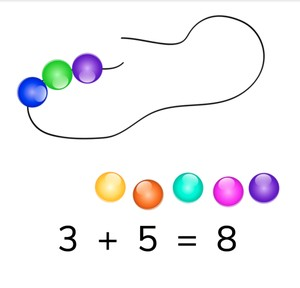# Recognizing addition problems to 10

I can recognize addition problems using pictures to 10.

No account needed.8,000 schools use Gynzy92,000 teachers use Gynzy1,600,000 students use Gynzy

## General

Students learn to use an image to help understand addition problems.

## Introduction

Show the image on the interactive whiteboard with different objects. The students must say how many of each object there are. Ask students if there are useful or quicker ways to count these objects. Say that you can cross off the counted objects. After the students answer, erase the grey box to check their answers.

## Instruction

Show the students the image of pencils on the interactive whiteboard. Some pencils are sharpened, others are unsharpened. First count the sharpened pencils, and then the pencils that are unsharpened. There are 5 sharpened pencils and 2 unsharpened pencils. The pencils that need to be sharpened are joining the sharpened pencils, they are being added. The addition problem is 5 + 2. Next, show 5 sharpened and 4 unsharpened pencils. That is 5 + 4. Discuss all the variations of pencils and show the addition problems. Next show the students the baby chicks. Say that you first count 6 baby chicks and then you count the second group, which only has 1 baby chick in the nest. Emphasize that the + means you add the two numbers together to create one larger number. Indicate that what you already have is the first number of the addition problem, and the objects that are added to it, are the second number.

Next show the field with dogs. Ask students which sum matches the image shown. Erase the grey boxes to check your answer. Next, they must drag the correct amount of goldfish to the aquarium. The addition problem is shown, and they need to make the image match the addition problem. Use the baby chicks to explain that if you know what the addition problem is, you can then solve for the sum, or total. Practice with the dishes to see if you can find the addition problem and answer to the image. Erase the grey square to check your answer.

Check that students understand recognizing addition problems with images to 10 by asking the following questions:
- How do you know which numbers are used in an addition problem?
- What must you use to show that you want to know the amount of both groups together?
- How do you know which number comes first in an addition problem?
- How do you know which number comes second in an addition problem?

## Quiz

Students first practice recognizing addition problems with visual support. Next, they must determine the addition problem and finally, they must also solve the addition problem.

## Closing

Discuss with students that it is important to recognize an addition problem and be able to solve them to 10. You need this to calculate quickly. Show students some statements on the board- two students answer an addition problem, but only one of them is correct. Students must determine which of the two is correct. Have students explain how they got to their answer.

## Teaching tips

Students who have difficulty adding using images can use MAB blocks. Set one group of blocks to the left, one to the right, and ask the student to count the blocks one by one.

## Instruction materials

MAB-blocks

### The online teaching platform for interactive whiteboards and displays in schools

• Save time building lessons

• Manage the classroom more efficiently

• Increase student engagement